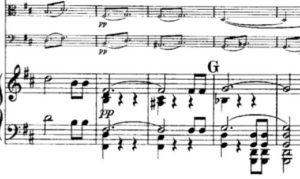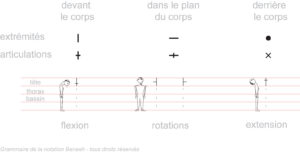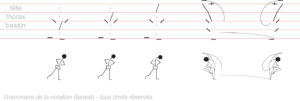# Some Cognizance notation systems

A system of notations is a set of graphic symbols, with rules of assembly and coherence, and in relation to a universe of knowledge and practices. There is an immense variety of notation systems, each adapted to its own universe.

## Examples of notation systems

• The mathematical notation :
• The first format of mathematicians was a’ literary/vocal’ coding: ” find a number such that the square multiplied by a second number to which the product of this first number is added by a third number and a constant is zero .
• The mathematical notation was gradually developed by the great mathematicians Euler, Leibniz, Newton, Lagrange, etc.
• ” a x² + bx + c = 0 ” is much shorter than the sentence above
• it is easy to recognize the similarity between ” a x² + bx + c = 0 ” and ” A + B y + C y² = 0 “
• the recognition of similarity is much more complex, if not impossible, between finding a number such that the square multiplied by a second number to which the product of this first number is added by a third number and a constant is zero . And find a number for which the sum of a constant, the product of this first number by a third number and finally the product of another number by the square of the first number is null.
• Mathematical notations are continuously evolving to translate new concepts.
• Musical notations , with their ranges, are another form of coding of knowledge.• The notion of emphasis is found with the notation of the musical volume (nuance): from pianissimo to fortissimo.
• Musical notation brings several concepts to traditional writing:
• the notion of parallelism : it is necessary to be able to play several notes at the same time.
• time management: tempo indications, duration of notes, durations of silences, etc.

• For dance , we can cite for example (among dozens of other systems) the Benesh notation, which codes the positions of the various parts of the body and whose principle is presented below:and an example below:• There are thus thousands of systems of notations for the communication each adapted to a universe:
• naval : flags of the boats, lights of the headlights, etc.
• chess : chess notation: 1.e4-e5, 2. d4!? – etc
• systems engineering : automatic diagrams
• Physics : Feynmann notations, etc.
• etc.

## The quality of a notation system:

Given the examples above, it is easy to understand the expected qualities of a good and efficient notation system:

• It must be compact :
• to minimize encoding and decoding processes
• in order to minimize the cognitive loads of use
• It must be simple to learn , to be able to be transmitted and used.
• It must be effective in the sense that the objects presented must be in simple relation with the objects to be communicated.

## Evolution of notation systems

The notation systems evolve according to a Darwinist principle towards conciseness and efficiency: for example the elegant Gothic writing has been gradually replaced by a cursive writing much simpler and faster to write.

## KM2: a new system of notations for knowledge

Traditional linear writing was invented by the Babylonians to transcribe discourses (intrinsically linear because of the time used by speech). But this writing is not a universal or practical mode for expressing knowledge: ideas are not connected linearly with one another. Basically, linear discourse is NOT the proper system of representation of knowledge, as we have just shown the above variety.

KM2 proposes a new system of notations: the cognitive schemes (or GHS notations), much better adapted) the representation of knowledge and processes. This change in notation must be accompanied by the tools that allow to manipulate these cognitive schemes efficiently and in large numbers, on an individual or team scale: the KM2 tools.